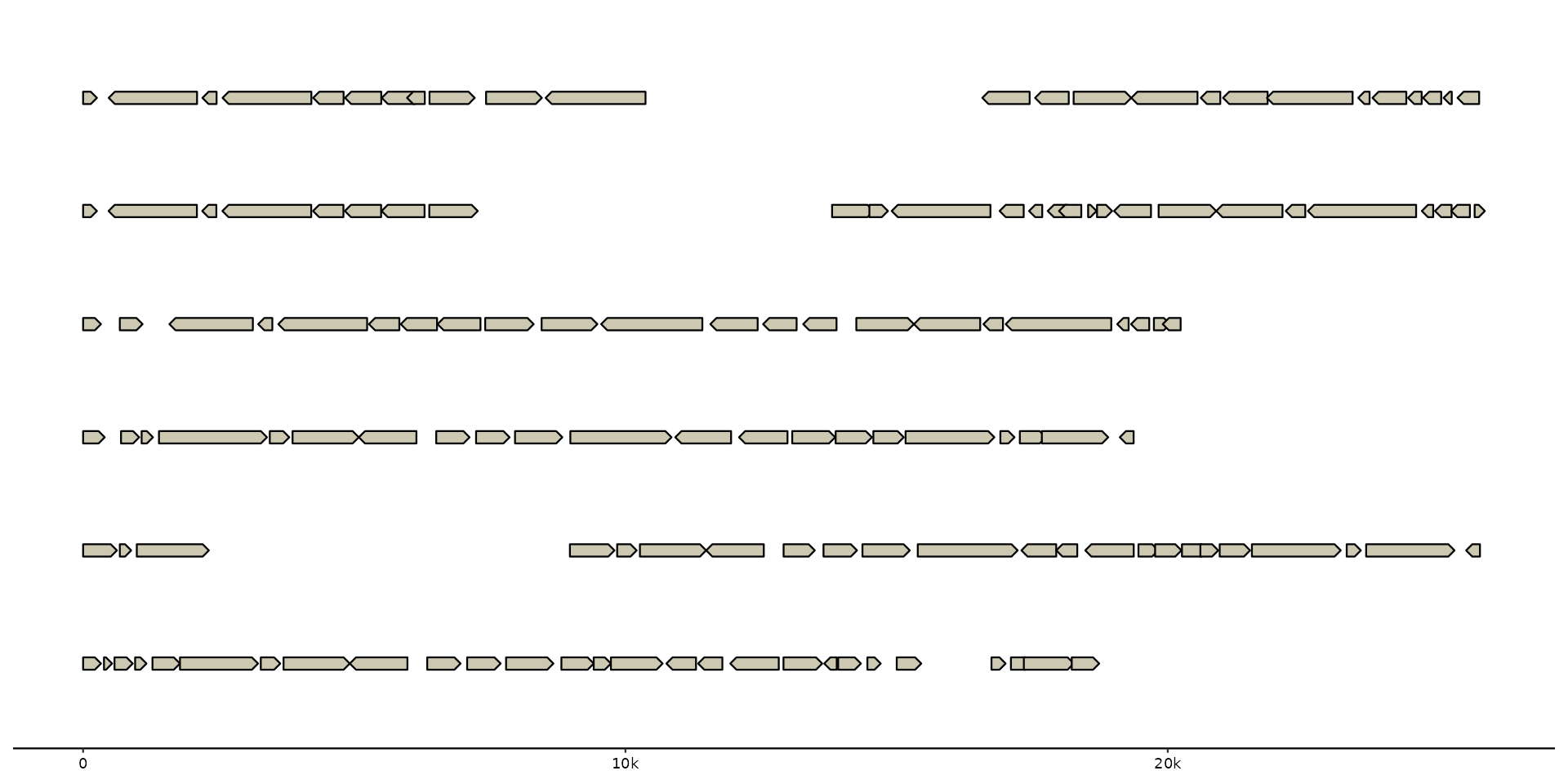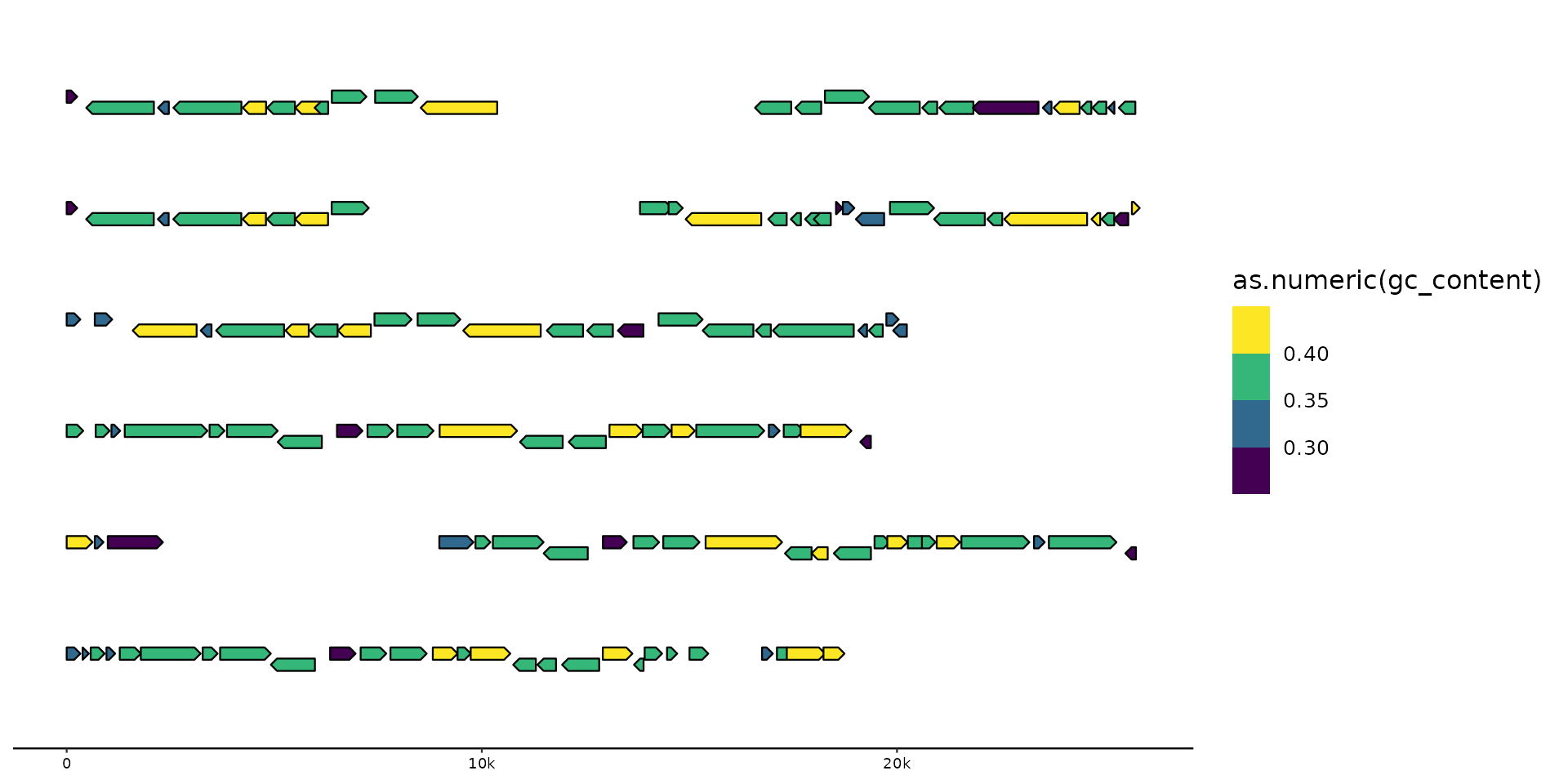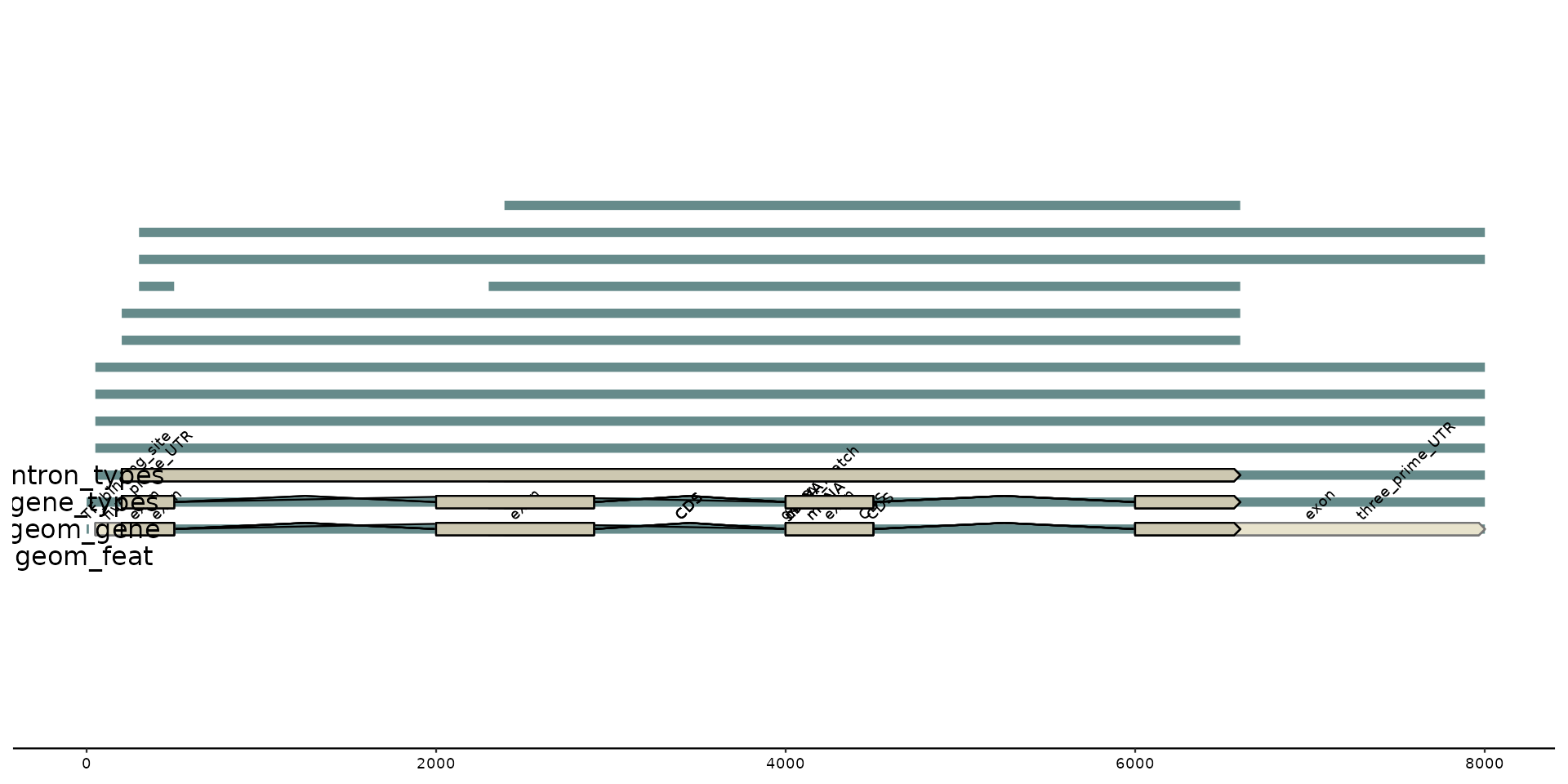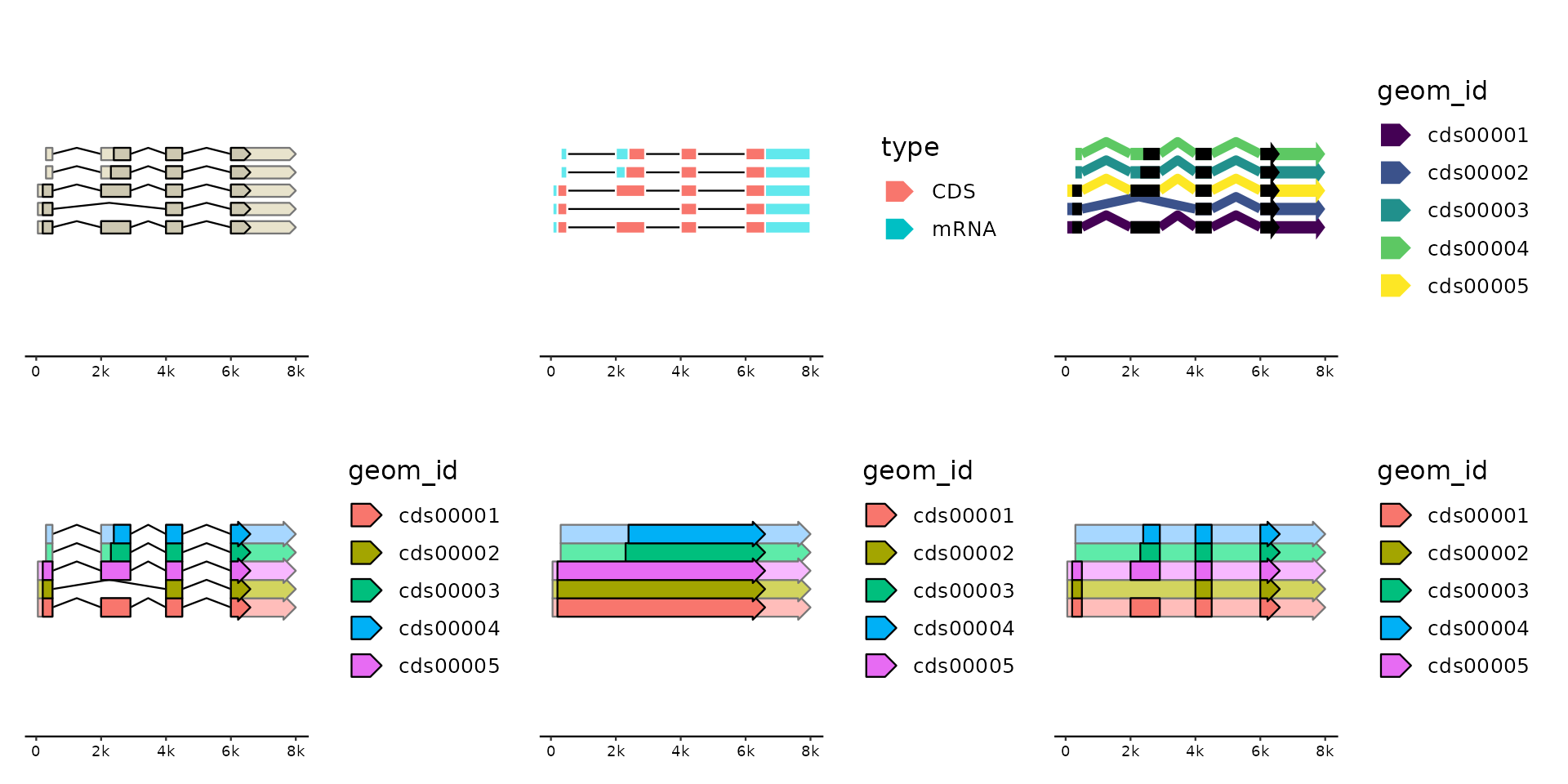Draw coding sequences, mRNAs and other non-coding features. Supports multi-exon features. CDS and mRNAs in the same group are plotted together. They can therefore also be positioned as a single unit using the position argument.

geom_gene(
mapping = NULL,
data = genes(),
stat = "identity",
position = "identity",
na.rm = FALSE,
show.legend = NA,
inherit.aes = TRUE,
size = 2,
rna_size = size,
shape = size,
rna_shape = shape,
intron_shape = size,
intron_types = c("CDS", "mRNA", "tRNA", "tmRNA", "ncRNA", "rRNA"),
cds_aes = NULL,
rna_aes = NULL,
intron_aes = NULL,
...
)

## Arguments

mapping

Set of aesthetic mappings created by aes() or aes_(). If specified and inherit.aes = TRUE (the default), it is combined with the default mapping at the top level of the plot. You must supply mapping if there is no plot mapping.

data

The data to be displayed in this layer. There are three options:

If NULL, the default, the data is inherited from the plot data as specified in the call to ggplot().

A data.frame, or other object, will override the plot data. All objects will be fortified to produce a data frame. See fortify() for which variables will be created.

A function will be called with a single argument, the plot data. The return value must be a data.frame, and will be used as the layer data. A function can be created from a formula (e.g. ~ head(.x, 10)).

stat

The statistical transformation to use on the data for this layer, as a string.

position

Position adjustment, either as a string, or the result of a call to a position adjustment function.

show.legend

logical. Should this layer be included in the legends? NA, the default, includes if any aesthetics are mapped. FALSE never includes, and TRUE always includes. It can also be a named logical vector to finely select the aesthetics to display.

inherit.aes

If FALSE, overrides the default aesthetics, rather than combining with them. This is most useful for helper functions that define both data and aesthetics and shouldn't inherit behaviour from the default plot specification, e.g. borders().

size, rna_size

the size of the gene model, aka the height of the polygons. rna_size only applies to non-coding parts of the gene model, defaults to size.

shape, rna_shape

vector of height and width of the arrow tip, defaults to size. If only one value is provided it is recycled. Set '0' to deactivates arrow-shaped tips. rna_shape only applies to non-coding parts of the gene model, defaults to shape.

intron_shape

single value controlling the kink of the intron line. Defaults to size. Set 0 for straight lines between exons.

intron_types

introns will only be computed/drawn for features with types listed here. Set to "CDS" to plot mRNAs as continous features, and set to NA to completely ignore introns.

cds_aes, rna_aes, intron_aes

overwrite aesthetics for different model parts. Need to be wrapped in ggplot2::aes(). NOTE: These remappings are applied after the data has been transformed and mapped by the plot scales (see ggplot2::after_scale()). So you need to map between aesthetic names (not data columns) and with standardized names, i.e. British English spelling. These mappings can be used to dynamically change parts of the gene model. For example, to change the color of introns from a hard-coded "black" to the same color used to fill the CDS you could specify intron_aes=aes(colour = fill). By default, rna_aes is remapped with aes(fill=colorspace::lighten(fill, .5), colour=colorspace::lighten(colour, .5)) to give it a lighter appearence than the corresponding CDS but in the same color.

## Aesthetics

geom_gene() understands the following aesthetics (required aesthetics are in bold):

• x

• xend

• y

• alpha

• colour

• fill

• group

• introns

• linetype

• stroke

• type

Learn more about setting these aesthetics in vignette("ggplot2-specs").

'type' and 'group' (mapped to 'type' and 'geom_id' by default) power the proper recognition of CDS and their corresponding mRNAs so that they can be drawn as one composite object. Overwrite 'group' to plot CDS and mRNAs independently.

'introns' (mapped to 'introns') is used to compute intron/exon boundaries. Use the parameter intron_types if you want to disable introns.

## Examples

gggenomes(genes=emale_genes) +
geom_gene()
#> No seqs provided, inferring seqs from featsgggenomes(genes=emale_genes) +
geom_gene(aes(fill=as.numeric(gc_content)),position="strand") +
scale_fill_viridis_b()
#> No seqs provided, inferring seqs from featstypes <- c(NA, "CDS", "mRNA", "tRNA", "tmRNA", "ncRNA", "rRNA", "intron",
"misc_RNA", "mobile_element", "operon", "...")
g0 <- tibble(seq_id="A", start=seq_along(types)*10,
end=seq_along(types)*10+7, type=types, introns=list(c(3,5)))

gggenomes(genes=g0) +
# all features in the "genes" regardless of type
geom_feat(data=feats(genes)) +
geom_text(aes(label="geom_feat", x=-15, y=.9)) + xlim(-20, NA) +
# only features in the "genes" of geneish type (implicit data=genes())
geom_gene() +
geom_gene_tag(aes(label=ifelse(is.na(type), "<NA>", type)), data=genes(.gene_types = NULL)) +
geom_text(aes(label="geom_gene", x=-15, y=1)) +
# control which types are returned from the track
geom_gene(aes(y=1.1), data=genes(.gene_types = c("CDS", "misc_RNA"))) +
geom_text(aes(label="gene_types", x=-15, y=1.1)) +
# control which types can have introns
geom_gene(aes(y=1.2, yend=1.2), data=genes(.gene_types = c("CDS", "misc_RNA")),  intron_types = "misc_RNA") +
geom_text(aes(label="intron_types", x=-15, y=1.2))
#> No seqs provided, inferring seqs from feats
#> Warning: Ignoring unknown aesthetics: yend# spliced genes
library(patchwork)
#> Harmonizing attribute names
#> • ID -> feat_id
#> • Name -> name
#> • Parent -> parent_ids
#> • Target -> target
#> # A tibble: 8 × 3
#>   source type                n
#>   <chr>  <chr>           <int>
#> 1 NA     CDS                 5
#> 2 NA     TF_binding_site     1
#> 3 NA     cDNA_match          1
#> 4 NA     exon                5
#> 5 NA     five_prime_UTR      1
#> 6 NA     gene                1
#> 7 NA     mRNA                5
#> 8 NA     three_prime_UTR     1
gg <- gggenomes(genes=g0)
#> No seqs provided, inferring seqs from feats
gg + geom_gene(position="pile") +
gg + geom_gene(aes(fill=type), position="pile",
shape = 0, intron_shape = 0, color="white") +
# some fine-control on cds/rna/intron after_scale aesthetics
gg + geom_gene(aes(fill=geom_id), position="pile",
size = 2, shape = c(4,3), rna_size = 2, intron_shape = 4, stroke=0,
cds_aes=aes(fill="black"), rna_aes=aes(fill=fill),
intron_aes=aes(colour=fill, stroke=2)) +
scale_fill_viridis_d() +
# fun with introns
gg + geom_gene(aes(fill=geom_id), position="pile", size = 3, shape=c(4,4)) +
gg + geom_gene(aes(fill=geom_id), position="pile", size = 3, shape=c(4,4),
intron_types=c()) +
gg + geom_gene(aes(fill=geom_id), position="pile", size = 3, shape=c(4,4),
intron_types="CDS")